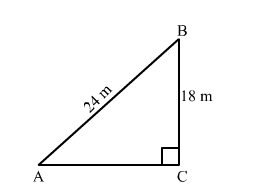# A guy wire attached to a vertical pole of height 18 m is 24 m long and has a stake attached to the other end.Question:

A guy wire attached to a vertical pole of height 18 m is 24 m long and has a stake attached to the other end. How far from the base of the pole should the stake be driven so that the wire will be taut?

Solution:Let AB be a guy wire attached to a pole BC of height 18 m. Now, to keep the wire taut let it to be fixed at A.
Now, In right triangle ABC
By using Pythagoras theorem, we have

$\mathrm{AB}^{2}=\mathrm{BC}^{2}+\mathrm{CA}^{2}$

$\Rightarrow 24^{2}=18^{2}+\mathrm{CA}^{2}$

$\Rightarrow \mathrm{CA}^{2}=576-324$

$\Rightarrow \mathrm{CA}^{2}=252$

$\Rightarrow \mathrm{CA}=6 \sqrt{7} \mathrm{~m}$

Hence, the stake should be driven $6 \sqrt{7} \mathrm{~m}$ far from the base of the pole.